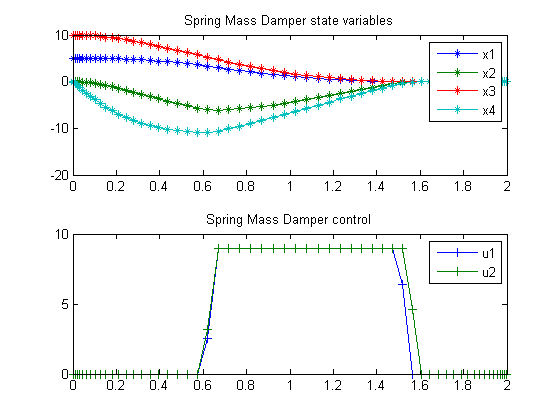107  Spring Mass Damper (2 Degree Freedom)

The Direct Approach of General Dynamic Optimal Control: Application on General Software

Tawiwat Veeraklaew, Ph.D. and Settapong Malisuwan, Ph.D. Chulachomklao Royal Military Academy Nakhon-Nayok, Thailand

107.1  Problem Formulation

Find u over t in [0; 2 ] to minimize

 J = u1+u2

subject to:

 dx dt
= A*x + B*u

Reference: 

107.2  Problem setup

toms t
p = tomPhase('p', t, 0, 2, 60);
setPhase(p);

tomStates x1 x2 x3 x4
tomControls u1 u2
x = [x1;x2;x3;x4];
u = [u1;u2];

m1 = 1.0; m2 = 1.0; c1 = 1.0; c3 = 1.0;
c2 = 2.0; k1 = 3.0; k2 = 3.0; k3 = 3.0;

B = [0 0; 1/m1 0;
0 0; 0 1/m2];

A = [0 1 0 0;...
1/m1*[-(k1+k2) -(c1+c2) k2 c2];...
0 0 0 1;...
1/m2*[ k2 c2 -(k2+k3) -(c2+c3)]];

x0i = [5; 0; 10; 0];
xfi = [0; 0;  0; 0];

% Box constraints
cbox = {0 <= collocate(u) <= 9};

% Boundary constraints
cbnd = {initial(x == x0i)
final(x == xfi)};

% ODEs and path constraints
ceq = collocate(dot(x) == A*x+B*u);

% Objective
objective = integrate(u1+u2);

107.3  Solve the problem

options = struct;
options.name = 'Spring Mass Damper';
solution = ezsolve(objective, {cbox, cbnd, ceq}, [], options);
t  = subs(collocate(t),solution);
x1 = subs(collocate(x1),solution);
x2 = subs(collocate(x2),solution);
x3 = subs(collocate(x3),solution);
x4 = subs(collocate(x4),solution);
u1 = subs(collocate(u1),solution);
u2 = subs(collocate(u2),solution);
Problem type appears to be: lp
Starting numeric solver
===== * * * =================================================================== * * *
TOMLAB - Tomlab Optimization Inc. Development license  999001. Valid to 2011-02-05
=====================================================================================
Problem: ---  1: Spring Mass Damper             f_k      16.485256203068737000
sum(|constr|)      0.000000008199340966
f(x_k) + sum(|constr|)     16.485256211268076000
f(x_0)      0.000000000000000000

Solver: CPLEX.  EXIT=0.  INFORM=1.
CPLEX Dual Simplex LP solver
Optimal solution found

FuncEv  243 Iter  243
CPU time: 0.093750 sec. Elapsed time: 0.094000 sec.

107.4  Plot result

subplot(2,1,1)
plot(t,x1,'*-',t,x2,'*-',t,x3,'*-',t,x4,'*-');
legend('x1','x2','x3','x4');
title('Spring Mass Damper state variables');

subplot(2,1,2)
plot(t,u1,'+-',t,u2,'+-');
legend('u1','u2');
title('Spring Mass Damper control');